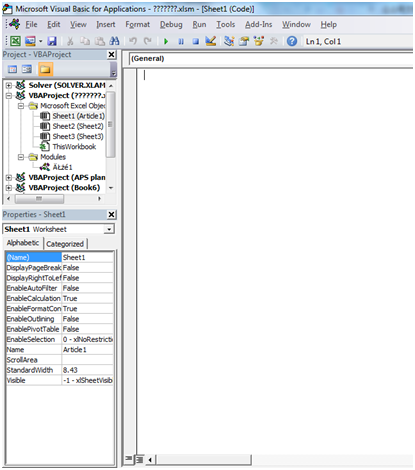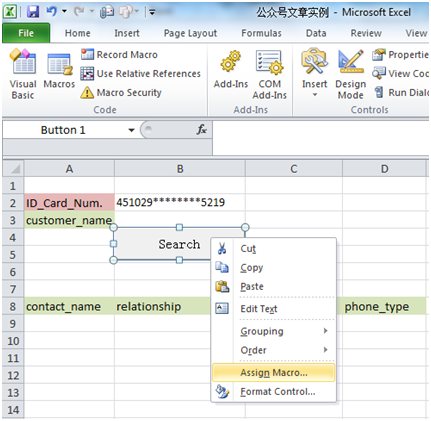top of page

# Introduction to database connections in Excel for efficient risk control – Part 4

Part 4: How to insert data from an excel sheet into a database tableIn previous articles we discussed how to connect to the database and download data in Excel. But what if you want to upload the data from excel into the database?

The requirement is that we will send the empty excel sheet to the person, he will fill in the sheet with the data and he clicks the button in the excel and the data has to be inserted into the database table. This solution should be used for not very large volumes of data uploaded, since the upload speed in this case is not very high. If you want to upload a large amount of information, you should use other tools.

We often use this approach when we need to provide a person who is not familiar (or not allowed) with using database directly with a tool for regularly storing important business data. In this article we present a simple example – upload list of call center employees.

Here, we use EXCEL2007 and Oracle SQL Server as the example. There might be some small differences between different versions.

STEP 1: Prepare the database table

In the database create a table into which data is inserted from Excel.In our example, the table is named ‘cl_operators’. We have 6 columns: MONTH, CITY, TEAM, NAME_TEAM_LEADER, NAME_OPERATOR, ID_OPERATOR.

STEP 2: Design Excel form for upload

1. Add the developer tab to Excel tool bar

We will use developer tab several times for our example, so let’s add it to tool bar first:

Click Office button -> EXCEL options -> Customized ribbon -> Developer -> AddFor our example, we design a table as below:This form includes the table for inserting data into and also some auxiliary fields.

Column G for each row contains the formula -=IF(E2>0;1;0), i.e. opposite each row will be 1 if the string contains data, and 0 if not.

Cell I1 contains the formula =SUM(G:G), which counts the number of data rows. Since initially we do not know how much data will added by user. We will use the value from cell I1to determine the range of data that we will add to the database in the future.

STEP 3: Connect database with VBA

1. Open VBA editor

Method 1: Alt + F11 (shortcut)

Method 2: (EXCEL tool bar) Developer -> Visual Basic:Before we write some VBA code, we need to add references to libraries (functions) that facilitate VBA running processes.

For different objects we used in our VBA code, we should add different references. For example, in our example, we use ADODB connection, so we should add corresponding ADODB library. Otherwise, we will get an error message when we run the VBA code because system doesn’t know how to deal with it.

(Tool bar) Tool -> Reference

For example, for Microsoft Active-X Data Objects, we have so many libraries as below:In our example, we add these references:3. Write VBA code according to the goal

Our code includes two parts.

The first part starts after pressing the 'upload' button: you simply define the data range that you want to insert and call the main procedure.

Sub Button_Click()

Call InsertData(Range("A2:F" &Cells(1, 9) + 1))

'Cells(1,9) value of cell I1

End Sub

In second part we use ADODB Connection.

Next is to create VBA code. Before we show the code, let’s explain the logic first. First we need to declare objects: ADODB connection (to connect to the database) and string objects which contains sql-code for inserting one row. Then we create connection to database and open the connection; then firstly, run the code that we want to execute before inserting the new data (in our example, firstly we delete the existing data for the same month that we want to add to avoid duplication of data); then use cycle FOR to write the result to database (for each row prepare the sql-code for insert data and then execute it); after finishing the work, we should close the connection.

Sub InsertData(rData As Range)

'declare connection

'declare string objects

Dim SQLString As String

Dim SQLStringH As String

Dim SQLStringV As String

Dim SQLStringBefore As String

Dim i As Long

Dim j As Long

Dim arrData()

arrData = rData.Value

If Cells(1, 9) > 0 Then'if value in cell I1>0, if range have some data

SQLStringH = "INSERT INTO CL_OPERATORS (MONTH,CITY,TEAM,NAME_TEAM_LEADER,NAME_OPERATOR,ID_OPERATOR) values ("

'define sql-code which will execute before inserting new data

SQLStringBefore = "DELETE FROM CL_OPERATORS WHERE MONTH=to_date('" & Cells(2, 1) & "','YYYY/MM/DD')"

'create connection to database

cn.ConnectionString = "Data Source=DWH;User ID=dtb;Password=111222333;Driver=(Oracle in OraClient12Home1);"

cn.Open

'execute SQLStringBefore

cn.ExecuteSQLStringBefore

'cycle is formed in which the sql code to add each row

For i = LBound(arrData, 1) To UBound(arrData, 1)

For j = LBound(arrData, 2) To UBound(arrData, 2)

If j < 6 Then

If j = 1 Then

SQLStringV = SQLStringV& "to_date('" &arrData(i, j) & "','YYYY/MM/DD'),"

Else

SQLStringV = SQLStringV& "'" &arrData(i, j) & "',"

End If

Else

SQLStringV = SQLStringV& "'" &arrData(i, j) & "')"

End If

Next j

SQLString = SQLStringH&SQLStringV

SQLStringV = Empty

'execute prepared sql-code for upload one row

cn.ExecuteSQLString

'move to next row

Next i

'Close connection to database

cn.Close

Set cn = Nothing

End If

End Sub

4. Connect ‘Upload’ button with macro

After writing the code need to assign macro code (first part of code – Sub ButtonClick()) with ‘upload’ button. We can do it like we did it in article 2:So, that’s all. Now we can put data to excel and add this information to database just by pushing our ‘upload’ button.

Tags:

bottom of page